Phet - chemistry; some simulations requires java plug in.

simulation has multiple potential lesson plans, which could be used as a potential inquiry group work activity and / or illustration during "lecture".

ch. 6 Electronic structure of atoms [Brown et al.]

 Beer's law: measure absorbance as a function of concentration, pathlength, & wavelength. if the java based simulation doesn't work, try the html5 version of the simulation.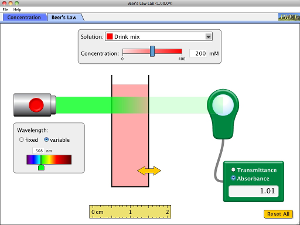Bohr model & spectroscopy: electron orbit transition as emission / absorption of light.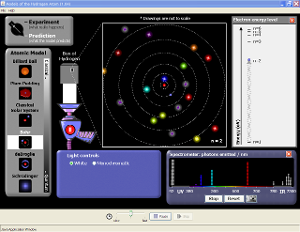photoelectric effect: illustrates Einstein's equation: E(incident) = W + KE.spectroscopy: animation somewhat crudely illustrate that different molecules absorb / emitt different type of light.

ch. 10 gas [Brown et al]

gas: measure T & P and histogram of speed & KE as a function of # gas molecules & heat / cool & gas mass.------------------------------------------------------------------------------------------------------------------------------------

ch. 11 modern atomic theory [Zumdahl et al.]

 Rutherford scattering: while there's a flaw in the simulation (i.e. inaccurate scale), it can illustrate the deflection of bombarding alpha particles by an atom's nucleus as a function of the alpha particles' KE.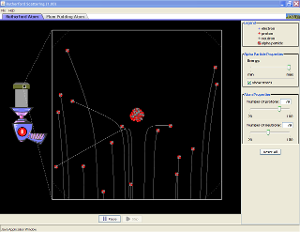atomic structure: relate (i) # protons, # neutrons, & mass #; (ii) charge & # electrons / protons; (iii) game related to preceding concepts. if the java based simulation doesn't work, try the html5 version of the simulation.isotope & average atomic mass: (i) stability of nucleus as a function of the # neutrons, (ii) isotope symbols, and (iii) average atomic mass based on relative amount of various isotopes.ch. 12 chemical bonding [Zumdhal et al.]

 VSEPR: show bond angles & shape of moleculesmolecular polarity: show dipole moment & electrostatic potential surfacech. 7 chemical equations [Zumdahl et al.]

 balancing chemical equation: shows molecules / atoms in chemical reaction; also, show atoms via balance or bar chart. there's a game in balancing chemical equations without prior bar chart or balance. if the java based simulation doesn't work, try the html5 version of the simulation.limiting reactant: uses sandwich example, 3 chemical equations with particulate diagrams, & games, where able to vary the level of "hints", which determines the level of difficulty.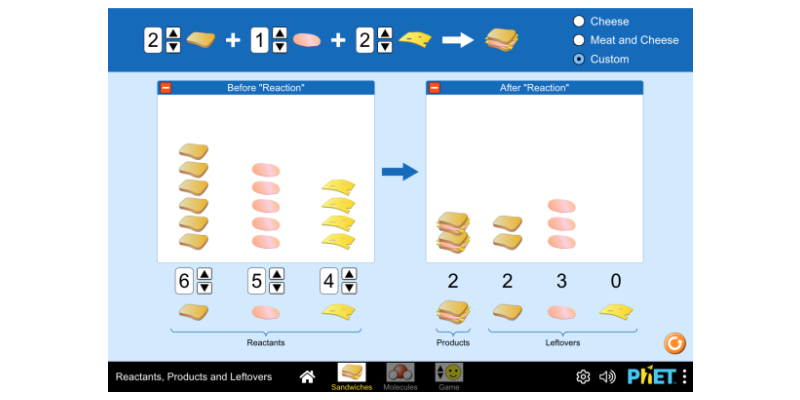ch. 5 measurement & calculation [Zumdahl et al.]

density: multiple simulations: (i) measure mass & volume of various objects to determine its density, (ii) same mass, but different volume, (iii) same volume, but different mass, and (iv) unknown object with table of density - to identify object. lab handoutch. 15 solutions [Zumdahl et al.]

 solution concentration: simulate effect on concentration by (i) addition of solute as solid or solution, and (ii) addition or removal of solvent. if the java based simulation doesn't work, try the html5 version of the simulation.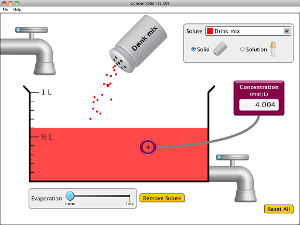solution: simulate various aspects of solution.macro tab: simulate effect of (i) dissolving solid salt or sugar and (ii) add / remove solvent on solution conductivity micro tab: simulate effect of (ii) adding different solid ionic compound or sugar and (ii) add / remove water on [solute] water tab: simulate hydration shell around ions and sugar in water. solution: simulate various aspect of solution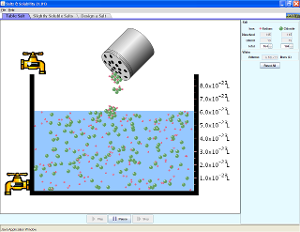tablet salt tab: show relative amount of dissolved versus undissolved species slightly soluble salt tab: similar to preceding simulation, but get a saturated solution quicker than preceding simulation design a salt: as preceding simulation, where able to simulate various Ksp values [probably inappropriate for regular chemistry class]

ch. 16 acid & base [Zumdahl et al]

 acid base: shows relative amount of acid / base, anion, & cation, as well as, simulate use of pH probe / pH paper / conductivity probe. able to simulate the effect of acid / base strength on the preceding parameters. if the java based simulation doesn't work, try the html5 version of the simulation.pH: simulate effect of adding water and acid / base on pH, [H +], & [OH -]. if the java based simulation doesn't work, try the html5 version of the simulation.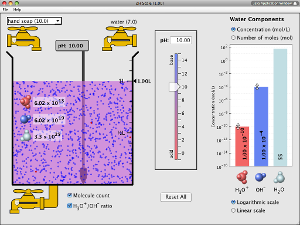blah blah --- pending evaluation

kinetics: blah blah -- pending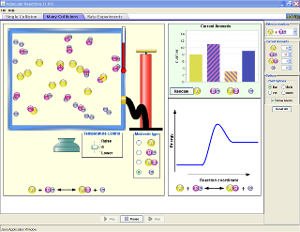deleted other simulations (since believe not "valuable"); not shown above

accessed Phet website on Oct 2014; so this website would not reflect any revision / addition / deletion of simulations after Oct 2014.

********************************************

based on somewhat superficial review of content at ACS Virtual chemistry & simulations website; access content on July 2020; so not reflect any revisions / addition / deletion after this date

AACT simulation / animation; access

 densitygas law simulationaverage atomic massGeneral Chemistry interactive simulations. very simplistic, not "engaging", but more convenient than me writing Excel simulations. access

 ionic solubilityspecific heat of metal IHess' lawspeed histogram: effect of temperature & molar massgas lawskinetics: graphical analysisupon reload webpage, changes rate law; believe error message is invalid Arrenihus equationthere's more, but . . .you explore

Bertrand's simulated experiments; access

seem to have terse directions, so maybe inappropriate for HS students without teacher support. feel free to explore. upon reload webpage, changes the problem

other  - misc simulations

CT interactive = links to misc simulations